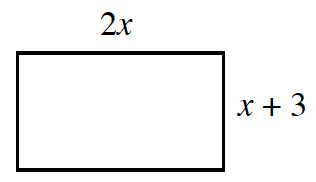Home > CC4 > Chapter 3 > Lesson 3.1.3 > Problem3-33

3-33.Examine the rectangle at right. Homework Help ✎

1. Write an expression for the perimeter in terms of $x$.

The perimeter is all of the sides added together, or $2l + 2w$.

2. Assuming the perimeter is $78$ cm, determine the dimensions of the rectangle. Show all of your work.

$6x + 6 = 78$
$6x = 72$
Solve for $x$ and then substitute for the $x$ in the expressions for the length and the width.

3. Verify that the area of this rectangle is $360$ sq. cm. Explain how you know this.

• In part (b), You found $x$. Now find the length and width.
The area is equal to the length times the width.